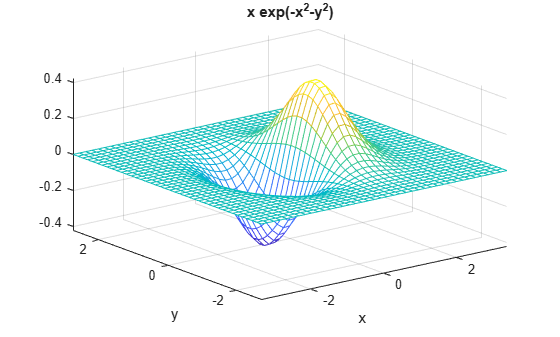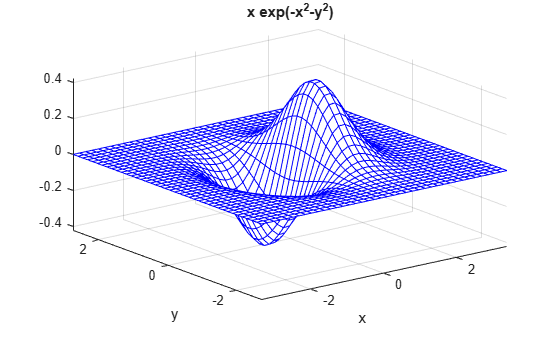# ezmesh

(Not recommended) Easy-to-use 3-D mesh plotter

`ezmesh` is not recommended. Use `fmesh` instead. For more information, see Compatibility Considerations.

## Syntax

``ezmesh(f)``
``ezmesh(f,xyinterval)``
``ezmesh(funx,funy,funz)``
``ezmesh(funx,funy,funz,uvinterval)``
``ezmesh(___,n)``
``ezmesh(___,'circ')``
``ezmesh(ax,___)``
``s = ezmesh(___)``

## Description

example

````ezmesh(f)` creates a mesh plot of the function `z = f(x,y)` using the `mesh` function. The function plots `f` over the default interval `[-2π 2π]` for `x` and `y`.`ezmesh` automatically adds a title and axis labels to the plot.```
````ezmesh(f,xyinterval)` plots over the specified interval. To use the same interval for both `x` and `y`, specify `xyinterval` as a two-element vector of the form `[min max]`. To use different intervals, specify a four-element vector of the form `[xmin xmax ymin ymax]`.```
````ezmesh(funx,funy,funz)` plots the parametric mesh defined by `x = funx(u,v)`, ```y = funy(u,v)```, `z = funz(u,v)` over the default interval `[-2π 2π]` for `u` and `v`.```
````ezmesh(funx,funy,funz,uvinterval)` plots the parametric mesh over the specified interval. To use the same interval for both `u` and `v`, specify `uvinterval` as a two-element vector of the form `[min max]`. To use different intervals, specify a four-element vector of the form `[umin umax vmin vmax]`.```
````ezmesh(___,n)` plots using an `n`-by-`n` grid. Use this option after any of the input argument combinations in the previous syntaxes.```
````ezmesh(___,'circ')` plots over a disk centered on the interval.```
````ezmesh(ax,___)` plots into the axes specified by `ax` instead of the current axes `gca`. Specify the axes before any of the input argument combinations in any of the previous syntaxes.```
````s = ezmesh(___)` returns the chart surface object. Use `s` to modify the mesh plot after it is created. For a list of properties, see Surface Properties.```

## Examples

collapse all

Create a mesh plot of the function $f\left(x,y\right)=x{e}^{-{x}^{2}-{y}^{2}}$ over a 40-by-40 grid.

```fh = @(x,y) x.*exp(-x.^2-y.^2); ezmesh(fh,40)```Set the mesh lines to a uniform blue color by setting the colormap to a single color.

`colormap([0 0 1])`## Input Arguments

collapse all

3-D function to plot, specified as a character vector, string scalar, or function handle to a named or anonymous function.

Specify a function of the form `z = f(x,y)`. The function must accept two matrix input arguments and return a matrix output argument of the same size.

When specifying the function as a character vector or string scalar, array multiplication, division, and exponentiation are always implied. For example, `x^2` is interpreted as `x.^2`.

Example: `'sqrt(x^2 + y^2)'`

When specifying the function as a function handle, use array operators instead of matrix operators for the best performance. For example, use `.*` (`times`) instead of * (`mtimes`).

Example: `@(x,y) sin(x).*cos(y)`

Plotting interval for `x` and `y`, specified in one of these forms:

• Vector of form `[min max]` — Use the interval `[min max]` for both `x` and `y`.

• Vector of form `[xmin xmax ymin ymax]` — Use the interval `[xmin xmax]` for `x` and `[ymin ymax]` for `y`.

Parametric function for x coordinates, specified as a character vector, string scalar, or function handle to a named or anonymous function.

Specify a function of the form `x = funx(u,v)`. The function must accept two matrix input arguments and return a matrix output argument of the same size.

When specifying the function as a character vector or string scalar, array multiplication, division, and exponentiation are always implied. For example, `x^2` is interpreted as `x.^2`.

Example: `'u*sin(v)'`

When specifying the function as a function handle, use array operators instead of matrix operators for the best performance. For example, use `.*` (`times`) instead of * (`mtimes`).

Example: `@(u,v) u.*sin(v)`

Parametric function for y coordinates, specified as a character vector, string scalar, or function handle to a named or anonymous function.

Specify a function of the form `y = funy(u,v)`. The function must accept two matrix input arguments and return a matrix output argument of the same size.

When specifying the function as a character vector or string scalar, array multiplication, division, and exponentiation are always implied. For example, `x^2` is interpreted as `x.^2`.

Example: `'-u.*cos(v)'`

When specifying the function as a function handle, use array operators instead of matrix operators for the best performance. For example, use `.*` (`times`) instead of * (`mtimes`).

Example: `@(u,v) -u.*cos(v)`

Parametric function for z coordinates, specified as a character vector, string scalar, or function handle to a named or anonymous function.

Specify a function of the form `z = funz(u,v)`. The function must accept two matrix input arguments and return a matrix output argument of the same size.

When specifying the function as a character vector or string scalar, array multiplication, division, and exponentiation are always implied. For example, `x^2` is interpreted as `x.^2`.

Example: `'-u*cos(v)'`

When specifying the function as a function handle, use array operators instead of matrix operators for the best performance. For example, use `.*` (`times`) instead of * (`mtimes`).

Example: `@(u,v) v`

Plotting interval for `u` and `v`, specified in one of these forms:

• Vector of form `[min max]` — Use the interval `[min max]` for both `u` and `v`.

• Vector of form `[umin umax vmin vmax]` — Use the interval `[umin umax]` for `u` and `[vmin vmax]` for `v`.

Size of the grid, specified as a positive integer. The grid has dimensions `n`-by-`n`.

Axes object. If you do not specify an axes object, then `ezmesh` uses the current axes.

## Version History

Introduced before R2006a

collapse all

### R2016a: `ezmesh` is not recommended

`ezmesh` is not recommended. Use `fmesh` instead. There are no plans to remove `ezmesh`.

`fmesh` requires that the input function to plot is a function handle. `ezmesh` accepts either a function handle, a character vector, or a string. This table shows some typical usages of `ezmesh` and how to update your code to use `fmesh` instead.

Not RecommendedRecommended
`ezmesh(@(x,y)x.*exp(-x.^2-y.^2),42)````fmesh(@(x,y) x.*exp(-x.^2-y.^2),'MeshDensity',42)```
`ezmesh('real(atan(x+i*y))')``fmesh(@(x,y) real(atan(x+i*y)))`
`ezmesh(@(x,y) sqrt(x.^2+y.^2))``fmesh(@(x,y) sqrt(x.^2+y.^2))`